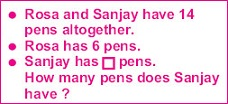# User ForumSubject :IMO    Class : Class 3

Ms Kajal wrote the problem as shown in the given box.Which number sentence matches the problem?

A+ 14 = 6
B- 14 = 6
C 14 -= 6
D 6 ×= 14

Rosa and Sanjay have 14 pens.Therefore Rosa Sanjay =14 pens.So, the answer is CRosa =6Sanjay=_The formula is 14-6=_14-6 =8Therefore S